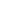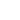AI

# 机器学习开放课程：三、分类、决策树和K近邻

【编者按】机器学习开放课程第三课，Mail.Ru数据科学家Yury Kashnitsky深入浅出地介绍了决策树、K近邻、交叉验证。#### 概览

1. 导言

2. 决策树

• 如何构建决策树
• 树构建算法
• 分类问题中的分割的其他质量标准
• 决策树如何应用于数值特征
• 决定性的树参数
• scikit-learn的`DecisionTreeClassifier`
• 回归问题中的决策树
3. 最近邻方法

• 实际应用中的最近邻方法
• scikit-learn的`KNeighborsClassifier`
4. 选择模型参数和交叉验证

5. 应用样例和复杂情形

• 客户离网率预测任务中的决策树和最近邻方法
• 决策树的复杂情形
• MNIST手写数字识别任务中的决策树和k-NN
• 最近邻方法的复杂情形
6. 决策树和最近邻方法的优势和劣势

7. 作业三

8. 相关资源

#### 1. 导言

• 基于特征分类实例为某类；
• 回归——预测实例的一个数值目标特征（基于实例的其他特征）；
• 聚类——基于实例的特征识别实例的分区，从而让组内成员更为相似（相比其他组的成员而言）；
• 异常侦测——寻找与其他样本或组内实例“很不一样”的实例；
• 其他更多。

Deep Learning（《深度学习》，Ian Goodfellow、Yoshua Bengio、Aaron Courville著，2016年出版）的“Machine Learning basics”（机器学习基础）一章提供了一份很好的综述。

#### 2. 决策树``````# 第一类
np.random.seed(17)
train_data = np.random.normal(size=(100, 2))
train_labels = np.zeros(100)

# 加入第二类
train_data = np.r_[train_data, np.random.normal(size=(100, 2), loc=2)]
train_labels = np.r_[train_labels, np.ones(100)]
``````

``````plt.rcParams['figure.figsize'] = (10,8)
plt.scatter(train_data[:, 0], train_data[:, 1], c=train_labels, s=100,
cmap='autumn', edgecolors='black', linewidth=1.5);
plt.plot(range(-2,5), range(4,-3,-1));
````````````from sklearn.tree import DecisionTreeClassifier

# 让我们编写一个辅助函数，返回之后的可视化网格
def get_grid(data):
x_min, x_max = data[:, 0].min() - 1, data[:, 0].max() + 1
y_min, y_max = data[:, 1].min() - 1, data[:, 1].max() + 1
return np.meshgrid(np.arange(x_min, x_max, 0.01),
np.arange(y_min, y_max, 0.01))
# max_depth参数限制树的深度。
clf_tree = DecisionTreeClassifier(criterion='entropy', max_depth=3,
random_state=17)
# 训练树
clf_tree.fit(train_data, train_labels)
# 可视化
xx, yy = get_grid(train_data)
predicted = clf_tree.predict(np.c_[xx.ravel(), yy.ravel()]).reshape(xx.shape)
plt.pcolormesh(xx, yy, predicted, cmap='autumn')
plt.scatter(train_data[:, 0], train_data[:, 1], c=train_labels, s=100,
cmap='autumn', edgecolors='black', linewidth=1.5);
````````````# 使用.dot格式可视化树
from ipywidgets import Image
from io import StringIO
import pydotplus #pip install pydotplus
from sklearn.tree import export_graphviz
dot_data = StringIO()
export_graphviz(clf_tree, feature_names=['x1', 'x2'],
out_file=dot_data, filled=True)
graph = pydotplus.graph_from_dot_data(dot_data.getvalue())
Image(value=graph.create_png())
````````````data = pd.DataFrame({'Age': [17,64,18,20,38,49,55,25,29,31,33],
'Loan Default': [1,0,1,0,1,0,0,1,1,0,1]})
# 让我们根据年龄进行升序排列
data.sort_values('Age')
``````

0 17 1
2 18 1
3 20 0
7 25 1
8 29 1
9 31 0
10 33 1
4 38 1
5 49 0
6 55 0
1 64 0
``````age_tree = DecisionTreeClassifier(random_state=17)
age_tree.fit(data['Age'].values.reshape(-1, 1), data['Loan Default'].values)
dot_data = StringIO()
export_graphviz(age_tree, feature_names=['Age'],
out_file=dot_data, filled=True)
graph = pydotplus.graph_from_dot_data(dot_data.getvalue())
Image(value=graph.create_png())
````````````data2 = pd.DataFrame({'Age':  [17,64,18,20,38,49,55,25,29,31,33],
'Salary': [25,80,22,36,37,59,74,70,33,102,88],
'Loan Default': [1,0,1,0,1,0,0,1,1,0,1]})
data2.sort_values('Age')
``````

``````age_sal_tree = DecisionTreeClassifier(random_state=17)
age_sal_tree.fit(data2[['Age', 'Salary']].values, data2['Loan Default'].values)
dot_data = StringIO()
export_graphviz(age_sal_tree, feature_names=['Age', 'Salary'],
out_file=dot_data, filled=True)
graph = pydotplus.graph_from_dot_data(dot_data.getvalue())
Image(value=graph.create_png())
``````

• 随机森林（一组树）将平均化构建到最大深度的单棵树的回应（我们以后会讨论为何要这么做）。

• 剪枝树。在这一方法中，树首先构建到最大深度。接着，从底部开始，通过比较有分区/无分区情形下树的质量，移除树的一些节点（比较基于交叉验证，下文会具体讨论）。• 人工限制深度或叶节点的最少样本数：达到限制时停止树的构造。

• 对树进行剪枝。

scikit-learn的`DecisionTreeClassifier`

`sklearn.tree.DecisionTreeClassifier`类的主要参数为：

• `max_depth` 树的最大深度；
• `max_features` 搜索最佳分区时的最大特征数（特征很多时，设置这个参数很有必要，因为基于所有特征搜索分区会很“昂贵”）；
• `min_samples_leaf` 叶节点的最少样本数。该参数防止创建任何叶节点只有很少成员的树。``````n_train = 150
n_test = 1000
noise = 0.1
def f(x):
x = x.ravel()
return np.exp(-x ** 2) + 1.5 * np.exp(-(x - 2) ** 2)
def generate(n_samples, noise):
X = np.random.rand(n_samples) * 10 - 5
X = np.sort(X).ravel()
y = np.exp(-X ** 2) + 1.5 * np.exp(-(X - 2) ** 2) + \
np.random.normal(0.0, noise, n_samples)
X = X.reshape((n_samples, 1))
return X, y
X_train, y_train = generate(n_samples=n_train, noise=noise)
X_test, y_test = generate(n_samples=n_test, noise=noise)
from sklearn.tree import DecisionTreeRegressor
reg_tree = DecisionTreeRegressor(max_depth=5, random_state=17)
reg_tree.fit(X_train, y_train)
reg_tree_pred = reg_tree.predict(X_test)
plt.figure(figsize=(10, 6))
plt.plot(X_test, f(X_test), "b")
plt.scatter(X_train, y_train, c="b", s=20)
plt.plot(X_test, reg_tree_pred, "g", lw=2)
plt.xlim([-5, 5])
plt.title("Decision tree regressor, MSE = %.2f" % np.sum((y_test - reg_tree_pred) ** 2))
plt.show()
``````#### 3. 最近邻方法1. 计算和训练集中每个样本的距离。

2. 从训练集中选取k个距离最近的样本。

3. 测试样本的分类将是它的k个最近邻中最常见的分类。

• 在某些案例中，k-NN可以作为一个开始（基线）；

• 在Kaggle竞赛中，k-NN常常用于构建元特征（即k-NN预测作为其他模型的输入），或用于堆叠/混合；

• 最近邻方法还可以用于其他任务，比如推荐系统。比如，推荐一项在接受推荐者的最近邻中很受欢迎的产品（或服务）；

• 实践中，在大型数据集上，常常使用逼近方法搜索最近邻。有不少实现这一算法的开源库；Spotify的Annoy了解下。

k-NN分类/回归的质量取决于一些参数：

• 邻居数k.

• 样本间距离的测量（常用的有Hamming、欧几里得、余弦、Minkowski距离）。注意大部分距离要求数据在同一尺度下。简单来说，我们不想让数量级以千计的“薪水”特征，影响通常小于100的“年龄”特征的距离。

• 邻居的权重（每个邻居可能贡献不同的权重；例如，样本越远，权重越低）。

scikit-learn的`KNeighborsClassifier`

`sklearn.neighbors.KNeighborsClassifier`类的主要参数为：

• `weights``uniform`（所有权重相等），`distance`（权重和到测试样本的距离成反比），或任何其他用户定义的函数；

• `algorithm`（可选）：`brute``ball_tree``KD_tree``auto``brute`，最近邻基于在训练集上的网格搜索得出。`ball_tree``KD_tree`，样本间的距离储存在树中，以加速寻找最近邻的过程。如果你设置该参数为`auto`，将基于训练集自动选择合适的寻找最近邻的方法。

• `leaf_size`（可选）：若寻找最近邻的算法是BallTree或KDTree，切换为网格搜索的阈值。

• `metric``minkowski``manhattan``euclidean``chebyshev`或其他。

#### 4. 选择模型参数和交叉验证

• 将数据集的一部分留置到一边（留置数据集）。我们保留一小部分训练集（一般是20%到40%），在剩余数据上训练模型（原数据集的60%-80%），然后在留置集上计算模型的表现度量（例如，精确度）。

• 交叉验证。最常见的情形是k折交叉验证#### 5. 应用样例和复杂情形

``````df = pd.read_csv('../../data/telecom_churn.csv')
df['International plan'] = pd.factorize(df['International plan'])
df['Voice mail plan'] = pd.factorize(df['Voice mail plan'])
df['Churn'] = df['Churn'].astype('int')
states = df['State']
y = df['Churn']
df.drop(['State', 'Churn'], axis=1, inplace=True)
````````````from sklearn.model_selection import train_test_split, StratifiedKFold
from sklearn.neighbors import KNeighborsClassifier
X_train, X_holdout, y_train, y_holdout = train_test_split(df.values, y,
test_size=0.3, random_state=17)
tree = DecisionTreeClassifier(max_depth=5, random_state=17)
knn = KNeighborsClassifier(n_neighbors=10)
tree.fit(X_train, y_train)
knn.fit(X_train, y_train)
``````

``````from sklearn.metrics import accuracy_score
tree_pred = tree.predict(X_holdout)
print(accuracy_score(y_holdout, tree_pred)) # 0.94
knn_pred = knn.predict(X_holdout)
print(accuracy_score(y_holdout, knn_pred)) # 0.88
``````

``````from sklearn.model_selection import GridSearchCV, cross_val_score
tree_params = {'max_depth': range(1, 11),
'max_features': range(4, 19)}
tree_grid = GridSearchCV(tree, tree_params,
cv=5, n_jobs=-1,
verbose=True)
tree_grid.fit(X_train, y_train)
``````

``````print(tree_grid.best_params_) # {'max_depth': 6, 'max_features': 17}
print(tree_grid.best_score_) # 0.942
print(accuracy_score(y_holdout, tree_grid.predict(X_holdout))) # 0.946
``````

``````dot_data = StringIO()
export_graphviz(tree_grid.best_estimator_, feature_names=df.columns,
out_file=dot_data, filled=True)
graph = pydotplus.graph_from_dot_data(dot_data.getvalue())
Image(value=graph.create_png())
````````````from sklearn.pipeline import Pipeline
from sklearn.preprocessing import StandardScaler
knn_pipe = Pipeline([('scaler', StandardScaler()),
('knn', KNeighborsClassifier(n_jobs=-1))])
knn_params = {'knn__n_neighbors': range(1, 10)}
knn_grid = GridSearchCV(knn_pipe, knn_params,
cv=5, n_jobs=-1, verbose=True)
knn_grid.fit(X_train, y_train)
print(knn_grid.best_params_, knn_grid.best_score_)
# ({'knn__n_neighbors': 7}, 0.886)
``````

``````from sklearn.ensemble import RandomForestClassifier

forest = RandomForestClassifier(n_estimators=100, n_jobs=-1,
random_state=17)
print(np.mean(cross_val_score(forest, X_train, y_train, cv=5))) # 0.949

forest_params = {'max_depth': range(1, 11), 'max_features': range(4, 19)}

forest_grid = GridSearchCV(forest, forest_params,
cv=5, n_jobs=-1, verbose=True)

forest_grid.fit(X_train, y_train)

print(forest_grid.best_params_, forest_grid.best_score_)
# ({'max_depth': 9, 'max_features': 6}, 0.951)
``````

``````def form_linearly_separable_data(n=500, x1_min=0, x1_max=30, x2_min=0, x2_max=30):
data, target = [], []
for i in range(n):
x1, x2 = np.random.randint(x1_min, x1_max), np.random.randint(x2_min, x2_max)
if np.abs(x1 - x2) > 0.5:
data.append([x1, x2])
target.append(np.sign(x1 - x2))
return np.array(data), np.array(target)
X, y = form_linearly_separable_data()
plt.scatter(X[:, 0], X[:, 1], c=y, cmap='autumn', edgecolors='black');
````````````tree = DecisionTreeClassifier(random_state=17).fit(X, y)
xx, yy = get_grid(X)
predicted = tree.predict(np.c_[xx.ravel(), yy.ravel()]).reshape(xx.shape)
plt.pcolormesh(xx, yy, predicted, cmap='autumn')
plt.scatter(X[:, 0], X[:, 1], c=y, s=100,
cmap='autumn', edgecolors='black', linewidth=1.5)
plt.title('Easy task. Decision tree complexifies everything');
````````````dot_data = StringIO()
export_graphviz(tree, feature_names=['x1', 'x2'],
out_file=dot_data, filled=True)
graph = pydotplus.graph_from_dot_data(dot_data.getvalue())
Image(value=graph.create_png())
````````````knn = KNeighborsClassifier(n_neighbors=1).fit(X, y)
xx, yy = get_grid(X)
predicted = knn.predict(np.c_[xx.ravel(), yy.ravel()]).reshape(xx.shape)
plt.pcolormesh(xx, yy, predicted, cmap='autumn')
plt.scatter(X[:, 0], X[:, 1], c=y, s=100,
cmap='autumn', edgecolors='black', linewidth=1.5);
plt.title('Easy task, kNN. Not bad');
``````MNIST手写数字识别任务中的决策树和k-NN

``````from sklearn.datasets import load_digits
data = load_digits()
X, y = data.data, data.target
f, axes = plt.subplots(1, 4, sharey=True, figsize=(16, 6))
for i in range(4):
axes[i].imshow(X[i,:].reshape([8,8]), cmap='Greys');
````````````X_train, X_holdout, y_train, y_holdout = train_test_split(X, y, test_size=0.3,
random_state=17)
``````

``````tree = DecisionTreeClassifier(max_depth=5, random_state=17)
knn = KNeighborsClassifier(n_neighbors=10)
tree.fit(X_train, y_train)
knn.fit(X_train, y_train)
``````

``````tree_pred = tree.predict(X_holdout)
knn_pred = knn.predict(X_holdout)
print(accuracy_score(y_holdout, knn_pred),
accuracy_score(y_holdout, tree_pred))
# (0.97, 0.666)
``````

``````tree_params = {'max_depth': [1, 2, 3, 5, 10, 20, 25, 30, 40, 50, 64],
'max_features': [1, 2, 3, 5, 10, 20 ,30, 50, 64]}
tree_grid = GridSearchCV(tree, tree_params, cv=5, n_jobs=-1,
verbose=True)
tree_grid.fit(X_train, y_train)
``````

``````print(tree_grid.best_params_, tree_grid.best_score_)
# ({'max_depth': 20, 'max_features': 64}, 0.844)
``````

``````print(np.mean(cross_val_score(KNeighborsClassifier(n_neighbors=1),
X_train, y_train, cv=5))) # 0.987
``````

``````print(np.mean(cross_val_score(RandomForestClassifier(random_state=17),
X_train, y_train, cv=5))) # 0.935
``````

kNN 0.987 0.983

``````def form_noisy_data(n_obj=1000, n_feat=100, random_seed=17):
np.random.seed(random_seed)
y = np.random.choice([-1, 1], size=n_obj)
# 第一个特征与目标成比例
x1 = 0.3 * y
# 其他特征为噪声
x_other = np.random.random(size=[n_obj, n_feat - 1])
return np.hstack([x1.reshape([n_obj, 1]), x_other]), y

X, y = form_noisy_data()
``````

``````X_train, X_holdout, y_train, y_holdout = train_test_split(X, y, test_size=0.3,
random_state=17)
from sklearn.model_selection import cross_val_score
cv_scores, holdout_scores = [], []
n_neighb = [1, 2, 3, 5] + list(range(50, 550, 50))
for k in n_neighb:
knn = KNeighborsClassifier(n_neighbors=k)
cv_scores.append(np.mean(cross_val_score(knn, X_train, y_train, cv=5)))
knn.fit(X_train, y_train)
holdout_scores.append(accuracy_score(y_holdout, knn.predict(X_holdout)))
plt.plot(n_neighb, cv_scores, label='CV')
plt.plot(n_neighb, holdout_scores, label='holdout')
plt.title('Easy task. kNN fails')
plt.legend();
````````````tree = DecisionTreeClassifier(random_state=17, max_depth=1)
tree_cv_score = np.mean(cross_val_score(tree, X_train, y_train, cv=5))
tree.fit(X_train, y_train)
tree_holdout_score = accuracy_score(y_holdout, tree.predict(X_holdout))
print(‘Decision tree. CV: {}, holdout: {}’.format(tree_cv_score,
tree_holdout_score))
# Decision tree. CV: 1.0, holdout: 1.0
``````

#### 6. 决策树和最近邻方法的优势和劣势

• 生成清晰、易于为人类理解的分类规则，例如“如果年龄不满25岁，并对摩托车感兴趣，拒绝发放贷款”。这一属性称为模型的可解释性。

• 决策树很容易可视化，即，模型本身（树）和特定测试对象的预测（穿过树的路径）可以“被解释”。

• 快速训练和预测。

• 较少参数数目。

• 支持数值和类别特征。

• 决策树对输入数据中的噪声非常敏感。这削弱了模型的可解释性。

• 决策树构建的边界有其局限性——它包含与坐标轴垂直的超平面，在实践中比其他方法的效果要差。

• 我们需要通过剪枝、设定叶节点的最小样本数、设定树的最大深度避免过拟合。注意所有机器学习方法都存在过拟合问题。

• 不稳定性。数据的小变动会显著改变决策树。这一问题可通过决策树集成处理（以后介绍）。

• 搜索最佳决策树是一个NP完全问题。实践中使用一些推断方法，比如基于最大信息增益进行贪婪搜索，但这并不能保证找到全局最优决策树。

• 难以支持数据中的缺失值。Friedman估计CART算法中大约50%的代码是为了支持数据中的缺口（`sklearn`实现了这一算法的改进版本）。

• 这一模型只能内插，不能外推（这一点同样适用于随机森林和树提升）。也就是说，对特征空间中，在由训练集圈定的包围盒之外的对象，决策树只能做出常数预测。在我们的黄球和蓝球的例子中，这意味着模型将对所有位于>19或<0的球做出同样的预测。

• 简单实现。

• 充分研究。

• 通常而言，这一方法不仅是分类或回归问题第一个值得尝试的选项，也是推荐问题中值得首先尝试的选项。

• 通过选择恰当的量度或核（一言以蔽之，核在k-NN方法的框架下为图之类的复杂对象设定了相似性运算），它可以适应特定问题。顺便提一下，以前在kaggle排名第一的Alexander Dyakonov偏爱最简单的k-NN算法（不过基于调整过的对象相似性量度）。

• 良好的可解释性。不过有例外：如果邻居的数目很大，可解释性会恶化（“我们没有给他发放贷款，因为他和350位客户类似，其中70位客户是不良客户，比数据集的平均值高12%”）。

• 和其他复合算法相比，这一方法算快的。但是，现实生活中，用于分类的邻居数目通常较大（100-150），在这一情形下，k-NN不如决策树快。

• 如果数据集有很多变量，很难找到合适的权重，也很难判定哪些特征对分类/回归不重要。

• 依赖于选择的对象间距离量度。默认选项欧几里得距离常常是不合理的。你可以通过网格搜索参数得到良好的解，但在大型数据集上这耗时很长。

• 没有理论方法选择邻居数——只能进行网格搜索（尽管对于所有模型的所有超参数而言，网格搜索常常是唯一方法）。在邻居数较小的情形下，该方法对离散值很敏感，也就是说，有过拟合的倾向。

• 由于“维度的诅咒”，有很多特征时它的表现并不好。ML社区知名成员Pedro Domingos教授，在他的流行论文“A Few Useful Things to Know about Machine Learning”中提及了这点。Ian Goodfellow等的《深度学习》第5章讨论了“纬度的诅咒”。

#### 8. 相关资源

• 每本ML书基本上都会介绍决策树和K近邻。我们推荐《Pattern Recognition and Machine Learning》（C. Bishop）和《Machine Learning: A Probabilistic Perspective》（K. Murphy）。

• 《Machine Learning in Action》（P. Harrington）将引导你完全使用Python实现经典ML算法。

• scikit-learn库。scikit-learn的开发者致力于编写极为清晰的文档。

• Scipy 2017 scikit-learn教程（Alex Gramfort、Andreas Mueller）。

• GitHub仓库rushter/MLAlgorithms里有许多ML算法的实现，其中包括决策树和k-NN。

• 欢迎留言分享其他资源。

### 评论游客2017-12-25

2017-12-29

2017-10-17

2017-11-10

### 文本数据分析（一）：基本框架发布需求联系客服